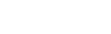# Video Analysis using OpenCV in Python

0
1348## IntroductionVideo Analysis using OpenCV in Python

In this article, We will explain the Video Analysis using OpenCV in Python not a video classification or not a object detection from video. We will learn about Meanshift and Camshift algorithms  to find and track objects in videos. It means you will learn the basic of video analysis how it is working and how its track the object.

There are two types of algorithms:

1. Meanshift
2. Camshift

Let’s explain in details

## Meanshift Algorithm

It is a basic algorithm. Consider you have a set of points.  (It can be a pixel distribution like histogram back projection). You are given a small window (may be a circle) and you have to move that window to the area of maximum pixel density (or maximum number of points). It is illustrated in the simple image given below:

The initial window is shown in blue circle with the name “C1”. Its original center is marked in blue rectangle, named “C1_o”. But if you find the centroid of the points inside that window, you will get the point “C1_r” (marked in small blue circle) which is the real centroid of window. Surely they do not match. So move your window such that circle of the new window matches with previous centroid. Again find the new centroid. Most probably, it will not match. So move it again, and continue the iterations such that center of window and its centroid falls on the same location (or with a small desired error). So finally what you obtain is a window with maximum pixel distribution. It is marked with green circle, named “C2”. As you can see in the image, it has maximum number of points. The whole process is demonstrated by a static image below:

### Meanshift Tutorial

To use mean shift in OpenCV, first, we need to set up the target, find its histogram so that we can back project the target on each frame for calculation of mean shift. We also need to provide the initial location of the window. For histogram, only Hue is considered here. Also, to avoid false values due to low light, low light values are discarded using cv2.inRange() function.

`````` Source Code:
import numpy as np
import cv2

cap = cv2.VideoCapture('slow.flv')

# take first frame of the video

# setup initial location of window
r,h,c,w = 250,90,400,125  # simply hardcoded the values
track_window = (c,r,w,h)

# set up the ROI for tracking
roi = frame[r:r+h, c:c+w]
hsv_roi =  cv2.cvtColor(frame, cv2.COLOR_BGR2HSV)
mask = cv2.inRange(hsv_roi, np.array((0., 60.,32.)), np.array((180.,255.,255.)))
cv2.normalize(roi_hist,roi_hist,0,255,cv2.NORM_MINMAX)

# Setup the termination criteria, either 10 iteration or move by atleast 1 pt
term_crit = ( cv2.TERM_CRITERIA_EPS | cv2.TERM_CRITERIA_COUNT, 10, 1 )

while(1):

if ret == True:
hsv = cv2.cvtColor(frame, cv2.COLOR_BGR2HSV)
dst = cv2.calcBackProject([hsv],,roi_hist,[0,180],1)

# apply meanshift to get the new location
ret, track_window = cv2.meanShift(dst, track_window, term_crit)

# Draw it on image
x,y,w,h = track_window
img2 = cv2.rectangle(frame, (x,y), (x+w,y+h), 255,2)
cv2.imshow('img2',img2)

k = cv2.waitKey(60) & 0xff
if k == 27:
break
else:
cv2.imwrite(chr(k)+".jpg",img2)

else:
break

cv2.destroyAllWindows()
cap.release()
``````## Camshift Algorithm

Did you closely watch the last result?(Meanshift Result) There is a problem. Our window (blue box) always has the same size when the car is farther away and it is very close to the camera. That is not good. We need to adapt the window size with size and rotation of the target. Once again, the solution came from “OpenCV Labs” and it is called CAMshift (Continuously Adaptive Meanshift) published by Gary Bradsky in his paper “Computer Vision Face Tracking for Use in a Perceptual User Interface” in 1988.

Widget not in any sidebars

It applies meanshift first. Once meanshift converges, it updates the size of the window as,It also calculates the orientation of the best fitting ellipse to it. Again it applies the meanshift with new scaled search window and previous window location. The process is continued until the required accuracy is met.

when you compare this two .gif file Camshift working is more accuracy with ellipse and find out distance.

### Camshift Tutorial

This algorithm almost work same as Meanshift, but camshift it detect the box (used to passed as search window in next iteration) and returns a rotated rectangle (i.e. main result).

Camshift code:

``````import numpy as np
import cv2

cap = cv2.VideoCapture('slow.flv')

# take first frame of the video

# setup initial location of window
r,h,c,w = 250,90,400,125  # simply hardcoded the values
track_window = (c,r,w,h)

# set up the ROI for tracking
roi = frame[r:r+h, c:c+w]
hsv_roi =  cv2.cvtColor(frame, cv2.COLOR_BGR2HSV)
mask = cv2.inRange(hsv_roi, np.array((0., 60.,32.)), np.array((180.,255.,255.)))
cv2.normalize(roi_hist,roi_hist,0,255,cv2.NORM_MINMAX)

# Setup the termination criteria, either 10 iteration or move by atleast 1 pt
term_crit = ( cv2.TERM_CRITERIA_EPS | cv2.TERM_CRITERIA_COUNT, 10, 1 )

while(1):

if ret == True:
hsv = cv2.cvtColor(frame, cv2.COLOR_BGR2HSV)
dst = cv2.calcBackProject([hsv],,roi_hist,[0,180],1)

# apply meanshift to get the new location
ret, track_window = cv2.CamShift(dst, track_window, term_crit)

# Draw it on image
pts = cv2.boxPoints(ret)
pts = np.int0(pts)
img2 = cv2.polylines(frame,[pts],True, 255,2)
cv2.imshow('img2',img2)

k = cv2.waitKey(60) & 0xff
if k == 27:
break
else:
cv2.imwrite(chr(k)+".jpg",img2)

else:
break

cv2.destroyAllWindows()
cap.release()

``````Now, observe that the difference between these results of two algorithms. Here, Camshaft is working on full frame.

## Conclusion

Widget not in any sidebars

Concluded that when working on video analysis or object detection most important is frame. So, out of two camshift is better working.